This function plots What-If Plots for a single prediction / observation.

# S3 method for ceteris_paribus_2d_explainer
plot(
x,
...,
facet_ncol = NULL,
bins = 3,
pch = "+",
size = 6
)

## Arguments

x a ceteris paribus explainer produced with the ceteris_paribus_2d() function currently will be ignored number of columns for the facet_wrap if TRUE then geom_raster will be added to present levels with diverging colors if TRUE then geom_contour will be added to present contours number of contours to be added if TRUE then geom_point will be added to present observation that is explained character, symbol used to plot observations numeric, size of individual datapoints

## Value

a ggplot2 object

Explanatory Model Analysis. Explore, Explain, and Examine Predictive Models. https://ema.drwhy.ai/

## Examples

library("DALEX")
library("ingredients")
library("ranger")

# \donttest{
apartments_rf_model <- ranger(m2.price ~., data = apartments)

explainer_rf <- explain(apartments_rf_model,
data = apartments_test[,-1],
y = apartments_test[,1],
verbose = FALSE)

new_apartment <- apartments_test[1,]
new_apartment
#>      m2.price construction.year surface floor no.rooms    district
#> 1001     4644              1976     131     3        5 Srodmiescie
wi_rf_2d <- ceteris_paribus_2d(explainer_rf, observation = new_apartment)
#>      y_hat new_x1 new_x2            vname1  vname2  label
#> 1 5222.393   1920   20.0 construction.year surface ranger
#> 2 5227.252   1920   21.3 construction.year surface ranger
#> 3 5247.392   1920   22.6 construction.year surface ranger
#> 4 5232.506   1920   23.9 construction.year surface ranger
#> 5 5242.054   1920   25.2 construction.year surface ranger
#> 6 5237.366   1920   26.5 construction.year surface ranger
plot(wi_rf_2d)
#> Warning: Raster pixels are placed at uneven horizontal intervals and will be shifted. Consider using geom_tile() instead.#> Warning: Raster pixels are placed at uneven vertical intervals and will be shifted. Consider using geom_tile() instead.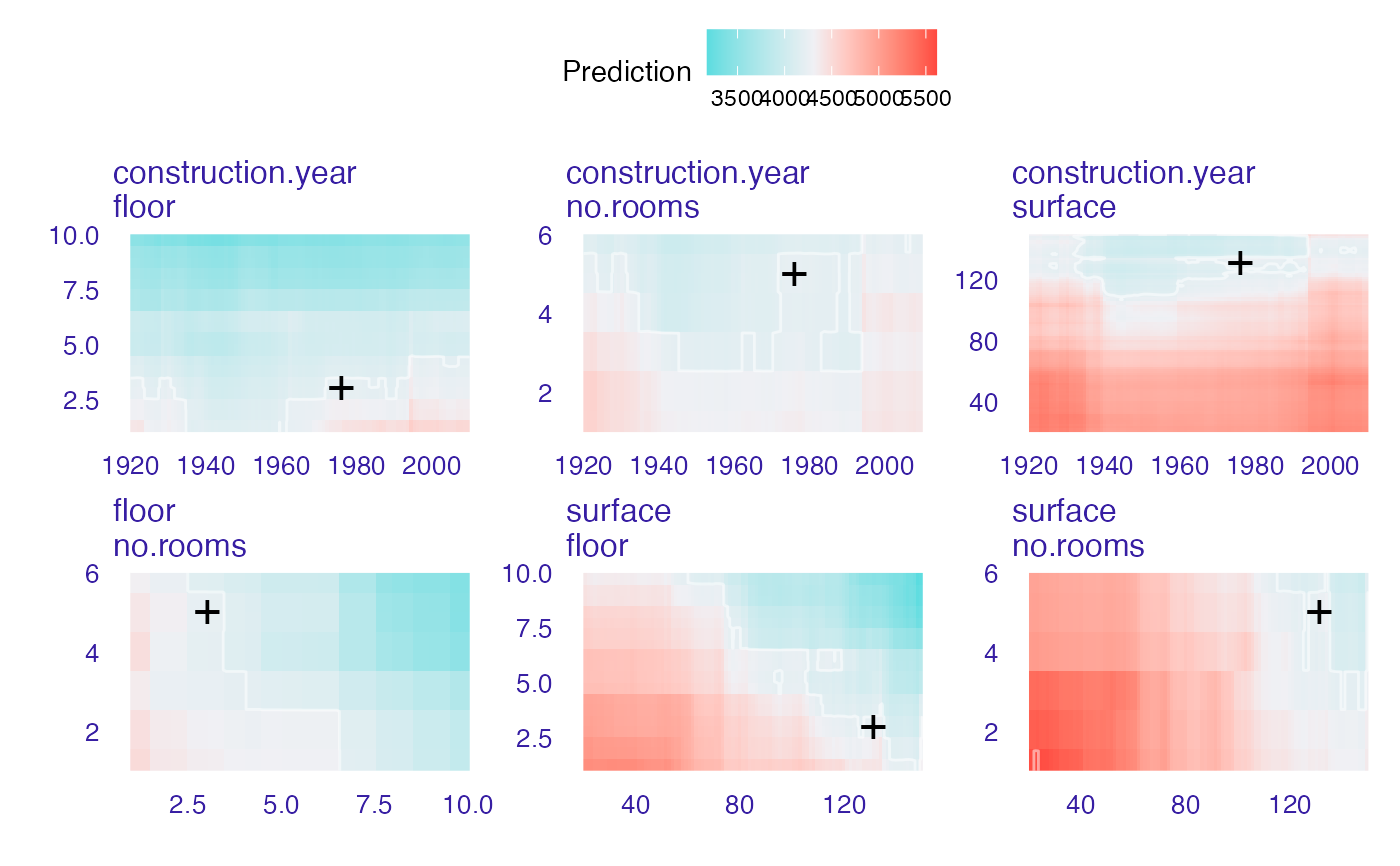plot(wi_rf_2d, add_contour = FALSE)
#> Warning: Raster pixels are placed at uneven horizontal intervals and will be shifted. Consider using geom_tile() instead.#> Warning: Raster pixels are placed at uneven vertical intervals and will be shifted. Consider using geom_tile() instead.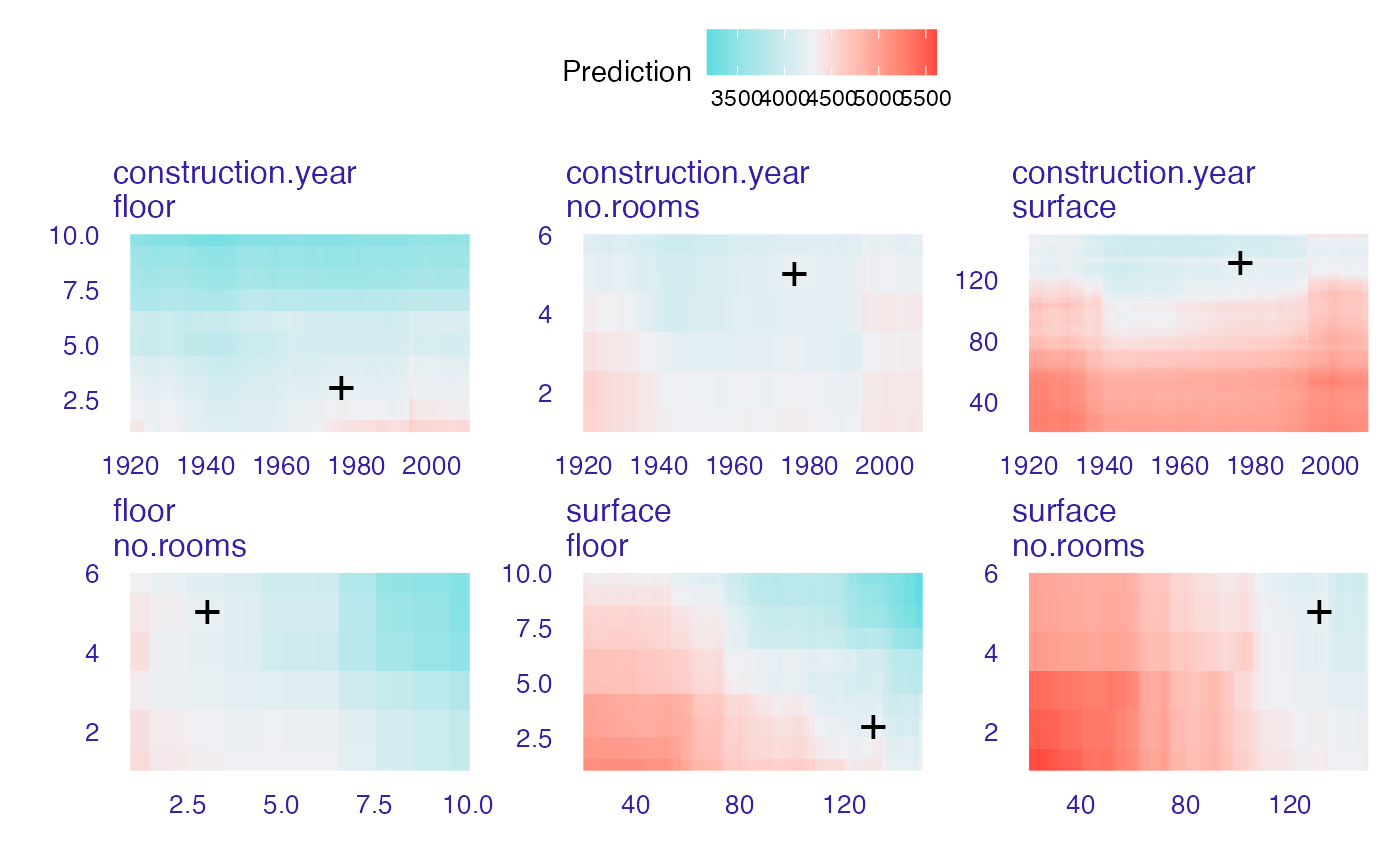plot(wi_rf_2d, add_observation = FALSE)
#> Warning: Raster pixels are placed at uneven horizontal intervals and will be shifted. Consider using geom_tile() instead.#> Warning: Raster pixels are placed at uneven vertical intervals and will be shifted. Consider using geom_tile() instead.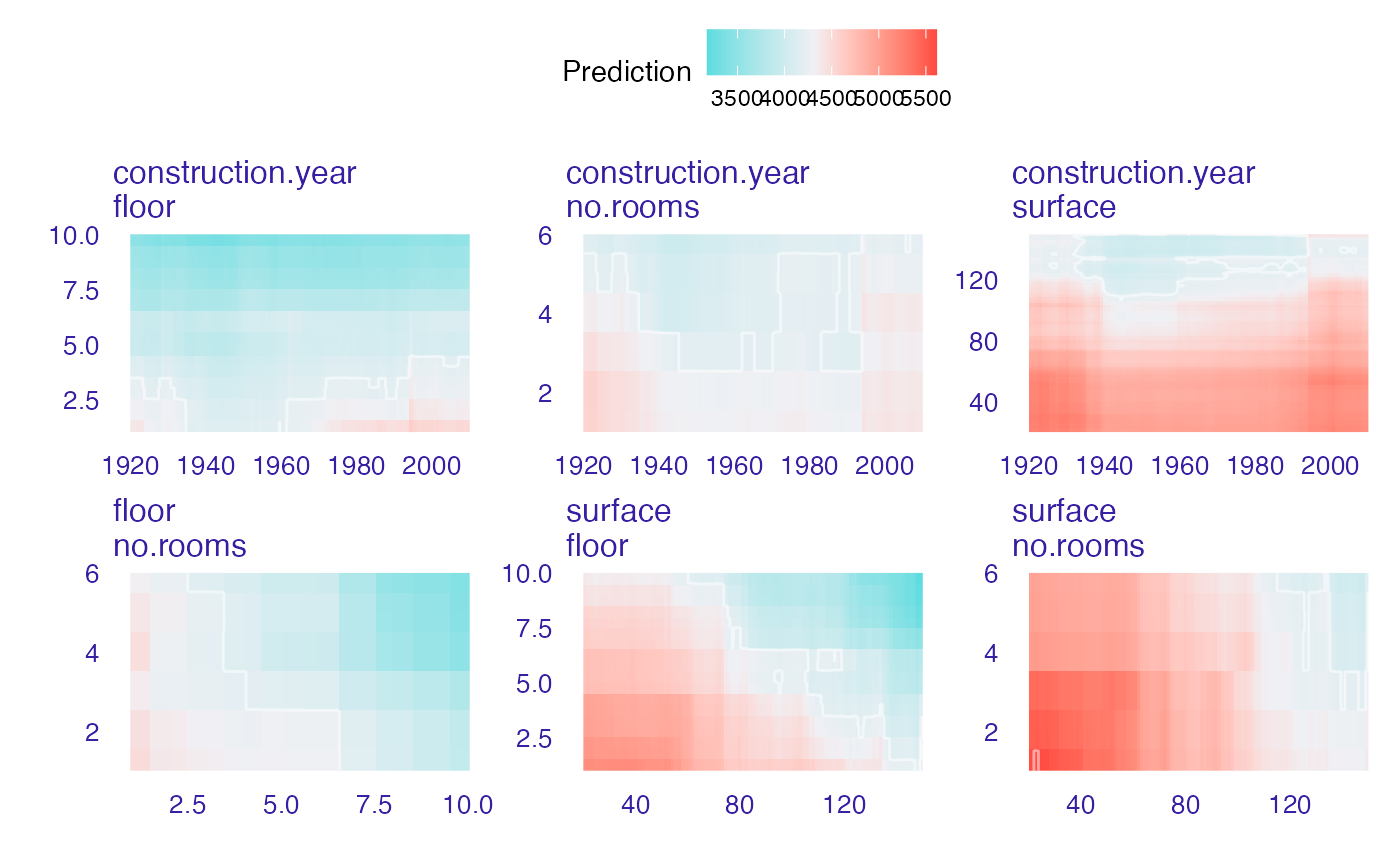plot(wi_rf_2d, add_raster = FALSE)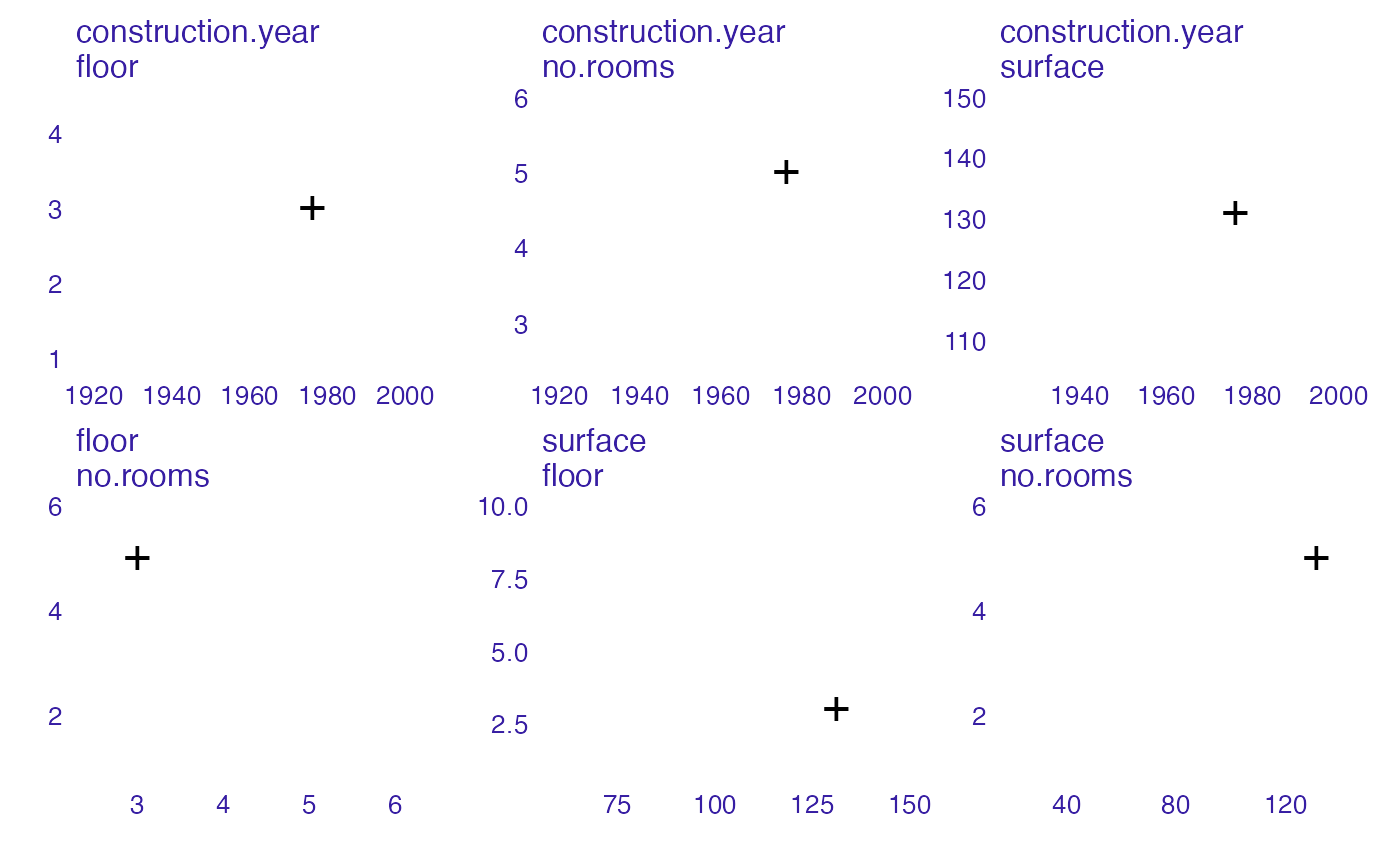# HR data
model <- ranger(status ~ gender + age + hours + evaluation + salary, data = HR,
probability = TRUE)

pred1 <- function(m, x)   predict(m, x)$predictions[,1] explainer_rf_fired <- explain(model, data = HR[,1:5], y = as.numeric(HR$status == "fired"),
predict_function = pred1,
label = "fired")
#> Preparation of a new explainer is initiated
#>   -> model label       :  fired
#>   -> data              :  7847  rows  5  cols
#>   -> target variable   :  7847  values
#>   -> predict function  :  pred1
#>   -> predicted values  :  No value for predict function target column. (  default  )
#>   -> model_info        :  package ranger , ver. 0.13.1 , task classification (  default  )
#>   -> predicted values  :  numerical, min =  0.0003492977 , mean =  0.3636236 , max =  0.9849971
#>   -> residual function :  difference between y and yhat (  default  )
#>   -> residuals         :  numerical, min =  -0.7439001 , mean =  0.0002096664 , max =  0.8139948
#>   A new explainer has been created!  new_emp <- HR[1,]
new_emp
#>   gender      age    hours evaluation salary status
#> 1   male 32.58267 41.88626          3      1  fired
wi_rf_2d <- ceteris_paribus_2d(explainer_rf_fired, observation = new_emp)
#> Warning: Raster pixels are placed at uneven horizontal intervals and will be shifted. Consider using geom_tile() instead.#> Warning: Raster pixels are placed at uneven vertical intervals and will be shifted. Consider using geom_tile() instead.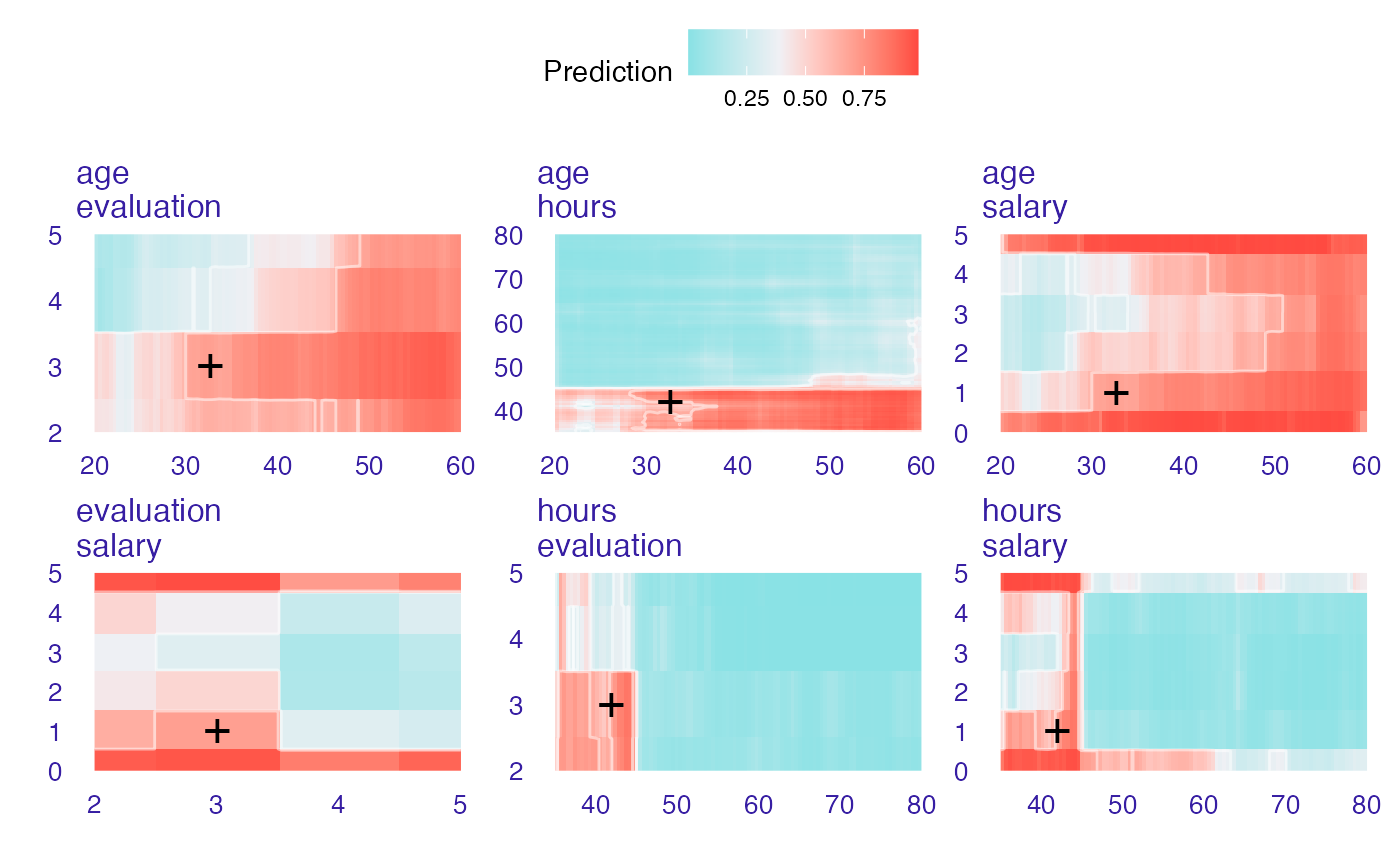# }